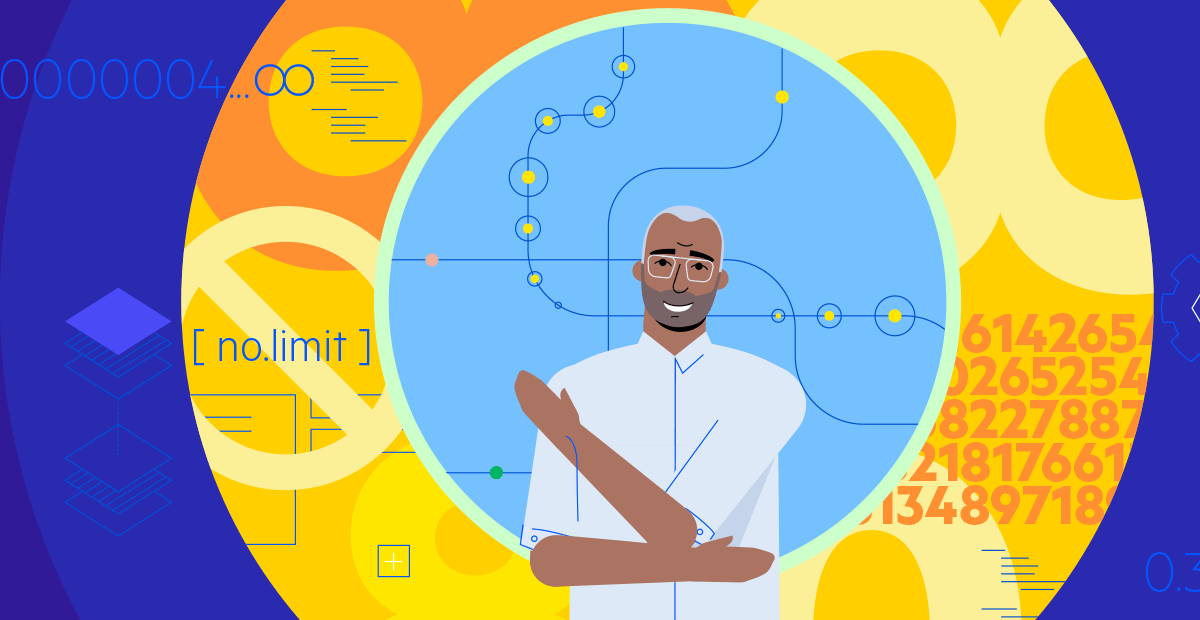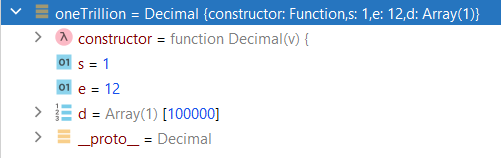Decimals With No Limitations in JavaScript

# Decimals With No Limitations in JavaScript

Posted on September 22, 2021You don’t want any errors in your commercial or Scientific applications, do you?

In the previous blog, we saw the issues we can run into when using the number type in JavaScript.

In this blog, we will see how using a decimal library can help alleviate those issues.

For example, Corticon.js uses a decimal library to implement its low-code/no-code decimal datatype. The library supports numbers with any precision, arbitrary small and large numbers and all typical mathematical operations, making it suitable for commercial and scientific applications.

In this blog, we will highlight the key characteristics of decimals. We will also go through the representation of decimals in JSON payload. And finally, we will explore what options you have for configuring decimals for maximum performance and if you need to deal with extremely large or small numbers.

## Limitations of the JavaScript Number Type

We saw in this blog that JavaScript numbers have some limitations that can be detrimental to scientific and commercial applications.

Here is a summary of these issues:

• Incorrect results with inexact numbers.
• Float computations are not deterministic.
• Very large or very small numbers cannot be processed, particularly when using integers.

Please refer to the blog for details.

## Decimal Library

A decimal library can help solve these issues. In Corticon.js, we leverage a great decimal library to implement our decimals datatype. The library is called decimal.js and you can find the doc here. It’s also available on Github at https://github.com/MikeMcl/decimal.js.

The key characteristic of this library and by extension, the Corticon.js Decimal datatype, is that they can represent and operate on numbers with any arbitrary precision. This allows for support of commercial and scientific applications, as we can do computations with arbitrary small or large numbers and select the precision needed.

## Corticon.js Decimals vs. JavaScript Number Type

Let’s highlight some differences between decimals in Corticon.js versus the number type in JavaScript.

We can do the following operations, for example, unlike using the JavaScript number, 0.1 + 0.2 is equal to 0.3. Or in a scientific application, one could multiply 123456789e+250 with 10e+150. It will yield the correct result 1.23456789e+409 (in JavaScript, these would exceed the maximum number).

So, how are there no limitations? Simply because you can configure the precision of numbers. The precision is a configuration parameter and is a key concept to understand.

What is it exactly? This parameter is the number of significant digits used to return results (that is the number of digits returned calculations are rounded to). See this link.

By default, this parameter is set to 20. Most of the time this default value will be sufficient, but in some cases, you may want to tune it based on your needs. The tradeoff is that computation time will increase with more precision digits. In other words, the higher the precision, the more digits you will have to represent numbers, but computations will take more CPU.

Don't be confused: The precision is not the number of decimal places. Instead, it is the number of significant digits used to express the returned numbers.

Let’s look at a couple of examples to see the effect and significance of the precision parameter.

We will use precision sets at 5. It's an unrealistic value for most cases but it is easier to understand the examples below.

### Example 1:

Rounding takes place for small numbers.

Decimal 0.123456789 multiplied by 1 will yield 0.12346 (5 is rounded up as it is followed by 6).

Decimal 0.123456789 plus 1000 will yield 1000.1 (with only 5 digits of precision we lose the fractional part 0.023456789).

### Example 2:

But large numbers can fit in 5 digits or less. For example:

Decimal 1,000,000,000,000 plus decimal 2,000,000,000,000 will yield the correct result 3,000,000,000,000.

That’s because these numbers can be expressed with 5 digits or less. For example, they could be expressed as 1 x 10e12 and 2 x 10e12. For both these numbers, we need just 3 digits: one for the number and 2 for the exponent.

For example, we can see the internal representation in the decimal.js library of the first number below using a debugger inspector:Here is one last example to show rounding with large numbers: Decimal 1,234,567,890,123 multiplied by Decimal 1 will yield 123460000000. Here, rounding takes place at the 5th digit.

There are multiple ways to round numbers. Check the last section on the configuration options for the rounding mode.

Corticon.js is used to implement low-code/no-code decision services. These services act on a JSON input payload and will return an output payload; as such, we need to understand how one can pass decimals to the decision service and what the expectations are for decimals returned in the output payload.

Corticon.js will accept and construct a decimal represented as either a number or a string, or as a string using exponent notation.

For example, the following is valid:

{ “cost”: 100.56, “cost2”: “100.56”, “cost3”: '10056e-2' }

The number or string representation should be helpful for commercial applications, while the exponent notation will be most helpful for scientific applications.

Decimals are always returned as strings in JSON, as the number type cannot represent all possible decimal values.

If you are working on a scientific application, you have the flexibility to force all numbers to be output with exponent notation, or you can adjust at what level the engine returns numbers with exponent notation. This is done using these 2 configuration elements:

• toExpPos: The positive exponent value at and above which toString returns exponential notation.
Note: when set to 0, exponent notation is always returned.
• toExpNeg: The negative exponent value at and below which toString returns exponential notation.
Note: when set to 0, exponent notation is always returned.

See the next section for a description of all the configurations options.

## Other Configuration Options

Here are additional configuration fields to control decimal operations:

The config has to be inserted in the main config object in field "decimal."

• precision: The maximum number of significant digits of the result of an operation.
number: integer, 1 to 1e+9 inclusive
Default value: 20
• rounding: The default rounding mode used when rounding the result of an operation to precision significant digits.
Default value: 4 (ROUND_HALF_UP)
0 Rounds away from zero
1 Rounds towards zero
2 Rounds towards Infinity
3 Rounds towards -Infinity
4 Rounds towards nearest neighbor. If equidistant, rounds away from zero
5 Rounds towards nearest neighbor. If equidistant, rounds towards zero
6 Rounds towards nearest neighbor. If equidistant, rounds towards even neighbor
7 Rounds towards nearest neighbor. If equidistant, rounds towards Infinity
8 Rounds towards nearest neighbor. If equidistant, rounds towards -Infinity
Rounding modes 0 to 6 (inclusive) are the same as those of Java's BigDecimal class.

Example:

 `const config = {` `decimal: {` `rounding: 6,` `precision: 50,` `toExpPos: 100,` `toExpNeg: -100` `}` ` ``... other configuration elements...` `}`

In conclusion, as we had seen in the previous blog, we can have computation issues with JavaScript numbers.

Corticon.js provides support for commercial and scientific applications using a robust decimal type that avoids issues with JavaScript numbers. It also has capabilities to express arbitrary small and large numbers, making it suitable for the most demanding commercial and scientific applications.## Thierry Ciot

Thierry Ciot is a Software Architect on the Corticon Business Rule Management System. Ciot has gained broad experience in the development of products ranging from development tools to production monitoring systems. He is now focusing on bringing Business Rule Management to Javascript and in particular to the serverless world where Corticon will shine. He holds two patents in the memory management space.

### Next:

Comments are disabled in preview mode.

#### Sitefinity Training and Certification Now Available.

Let our experts teach you how to use Sitefinity's best-in-class features to deliver compelling digital experiences.

##### More From Progress##### Latest Stories in Your Inbox

Subscribe to get all the news, info and tutorials you need to build better business apps and sites